Name:    Chapter 3 Test

Multiple Choice
Identify the choice that best completes the statement or answers the question.

Estimate by rounding each factor to the nearest whole number.

1.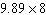a. 72 c. 79.2 b. 88 d. 80

2.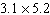a. 16 c. 15 b. 9 d. 21

Estimate the area of the figure by rounding to the nearest whole number.

3.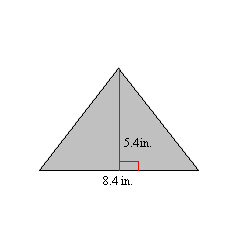a. 20.5 in.2 c. 28 in.2 b. 24 in.2 d. 20 in.2

Use mental math to find the product.

4.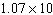a. 1070 c. 107 b. 0.107 d. 10.7

Use the Distributive Property to rewrite the expression.

5.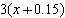a. 3x + 0.45 c. 3x + 0.15 b. 3.15x d. 0.45x

6.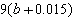a. 9b + 0.015 c. 9.015b b. 0.135b d. 9b + 0.135

Evaluate the expression.

7.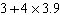a. 18.6 c. 15.9 b. 15.7 d. 27.3

8.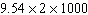a. 9,542 c. 11,540 b. 19,080 d. 1,019.1

Evaluate the expression when x = 1.4 and y = 4.21.

9.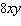a. 4.7152 c. 47.152 b. 11.2 d. 4.7252

10.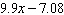a. 34.699 c. 6.79 b. 34.599 d. 6.78

11.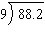a. 9.7 c. 98.1 b. 9.8 d. 98

12.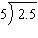a. 5 c. 0.5 b. 5.1 d. 0.4

13.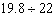a. 9.1 c. 9 b. 0.9 d. 0.8

14.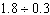a. 60 c. 5.9 b. 6.1 d. 6

15.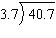a. 11.1 c. 10.9 b. 110 d. 11

16.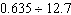a. 0.05 c. 0.06 b. 0.5 d. 0.04

Numeric Response

1.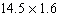2.

A Chinese restaurant offers buffet takeout for \$6.73 per pound. How much does your take out meal cost if your meal weighs 5.78 pounds?

Tell wether you would use rounding or compatible numbers to estimate the product or quotient. Explain your reasoning.

1.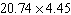2.

The weight of an object on Jupiter is about 2.36 of its weight on Earth. How much does a 230 pound astronaut weigh on Jupiter?